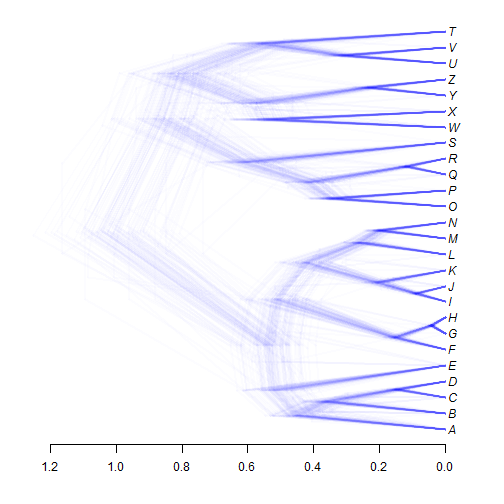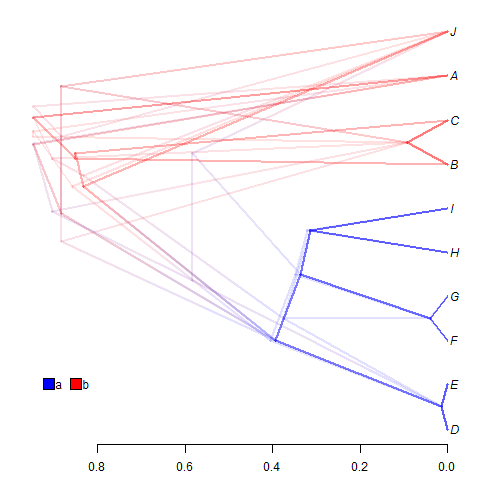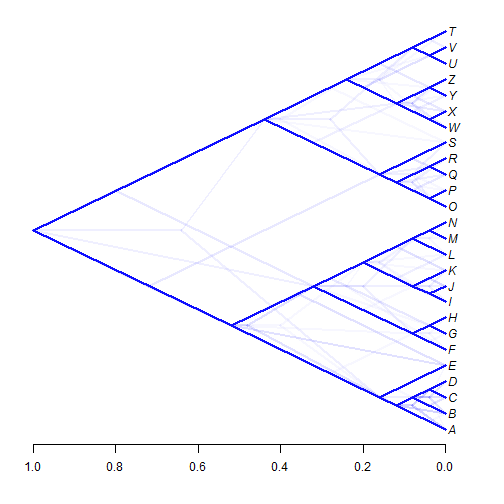## Thursday, April 27, 2017

### Slanted phylogram & cladogram `densityTree` plotting using phytools

Adding a slanted phylogram (aka. 'cladogram') method to `plotSimmap`, as I did yesterday, had the unintented but immediate effect of permitting us to very closely approximate the slanted tree style of both the original DensiTree software & Klaus Schliep's `densiTree` function of the phangorn R package.

Here is what that looks like using phytools:

``````library(phytools)
packageVersion("phytools")
``````
``````##  '0.6.11'
``````
``````tt
``````
``````## 99 phylogenetic trees
``````
``````densityTree(tt,type="cladogram",nodes="intermediate")
``````We can do the same with stochastic map trees. For instance:

``````cols<-setNames(c("blue","red"),c("a","b"))
nodes="intermediate",fix.depth=TRUE)
``````
``````add.simmap.legend(colors=cols,prompt=FALSE,x=0.9,y=2,vertical=FALSE)
````````````##  TRUE
``````

We need to set the node position to `"intermediate"` because the default for `densityTree`, as I mentioned in a previous post, is `"inner"` - but this works much better for a square phylogram than a slanted one!

Note that I also had to fix `add.simmap.legend` to permit the legend to plot correctly when our axis is flipped, as is done for `densityTree` plots.

We can also show just the topological incongruence among trees as follows:

``````densityTree(tt,use.edge.length=FALSE,type="cladogram",nodes="centered",
alpha=0.03)
``````For this one, I used `nodes="centered"` and increaed the `alpha` transparency level over its default (1/N) simply because there is so little topological incongruence in our set of trees that it is otherwise hard to see.

That's it for now.